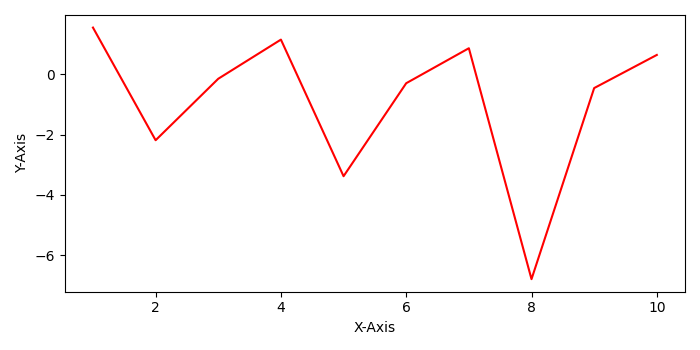# How to get the list of axes for a figure in Pyplot?

To get a list of axes of a figure, we will first create a figure and then, use get_axes() method to get the axes and set the labels of those axes.

• Create xs and ys using numpy and fig using figure() method. Create a new figure, or activate an existing figure.
• Use add_subplot() method. Add an '~.axes.Axes' to the figure as part of a subplot arrangement, where nrows=1, ncols=1 and index=1.
• . Get the axes of the fig, and set the xlabel and ylabel.
• Plot x and y data points with red color.
• To display the figure, use show() method.

## Example

import numpy as np
from matplotlib import pyplot as plt
plt.rcParams["figure.figsize"] = [7.00, 3.50]
plt.rcParams["figure.autolayout"] = True
xs = np.linspace(1, 10, 10)
ys = np.tan(xs)
fig = plt.figure()
plt.show()